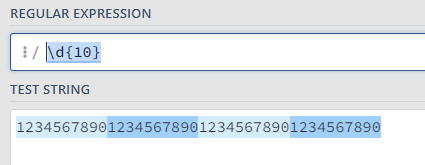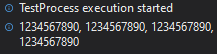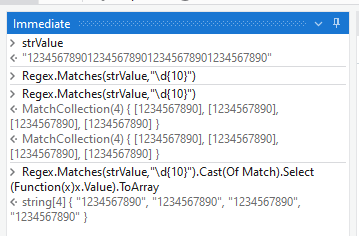# Cut long lines of numbers by 10

hello

I want to cut the long stretched numbers by 10.

1234567890123456789012345678901234567890

=> 1234567890, 1234567890, 1234567890,1234567890

Like this!
What should I do?

Hi @bbbb,

You can use a Matches activity with the following Regex pattern:

`\d{10}`Edit: This will give you an array of matches, if you need them in one string like you’ve specified then use the following:

`String.Join(", ",YourMatches)`Hi,
cutNumbers = Enumerable.Range(0, number.Length / 10).Select(Function(i) number.Substring(i * 10, 10)).ToList()
Now you can use the “Write Line” activity to display the contents of the “cutNumbers” list. Place this activity inside a loop to print each chunk separately.
For Each item In cutNumbers
Write Line activity: item
Next
Thank you

Hi @bbbb ,

With the Expression method :

``````Regex.Matches(strValue,"\d{10}").Cast(Of Match).Select(Function(x)x.Value).ToArray
``````Here, the assumption is you would require each 10 numbers as an item in the array.

1 Like

Hello you can use Regex do to that.

TestSplit.xaml (6.2 KB)

when it is not guaranteed that the string length is always a multiple of 10 then we can handle this felxible as done below:

2 Likes